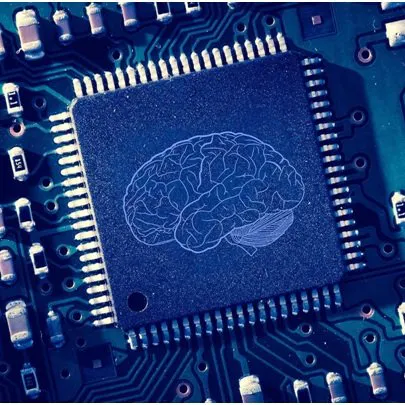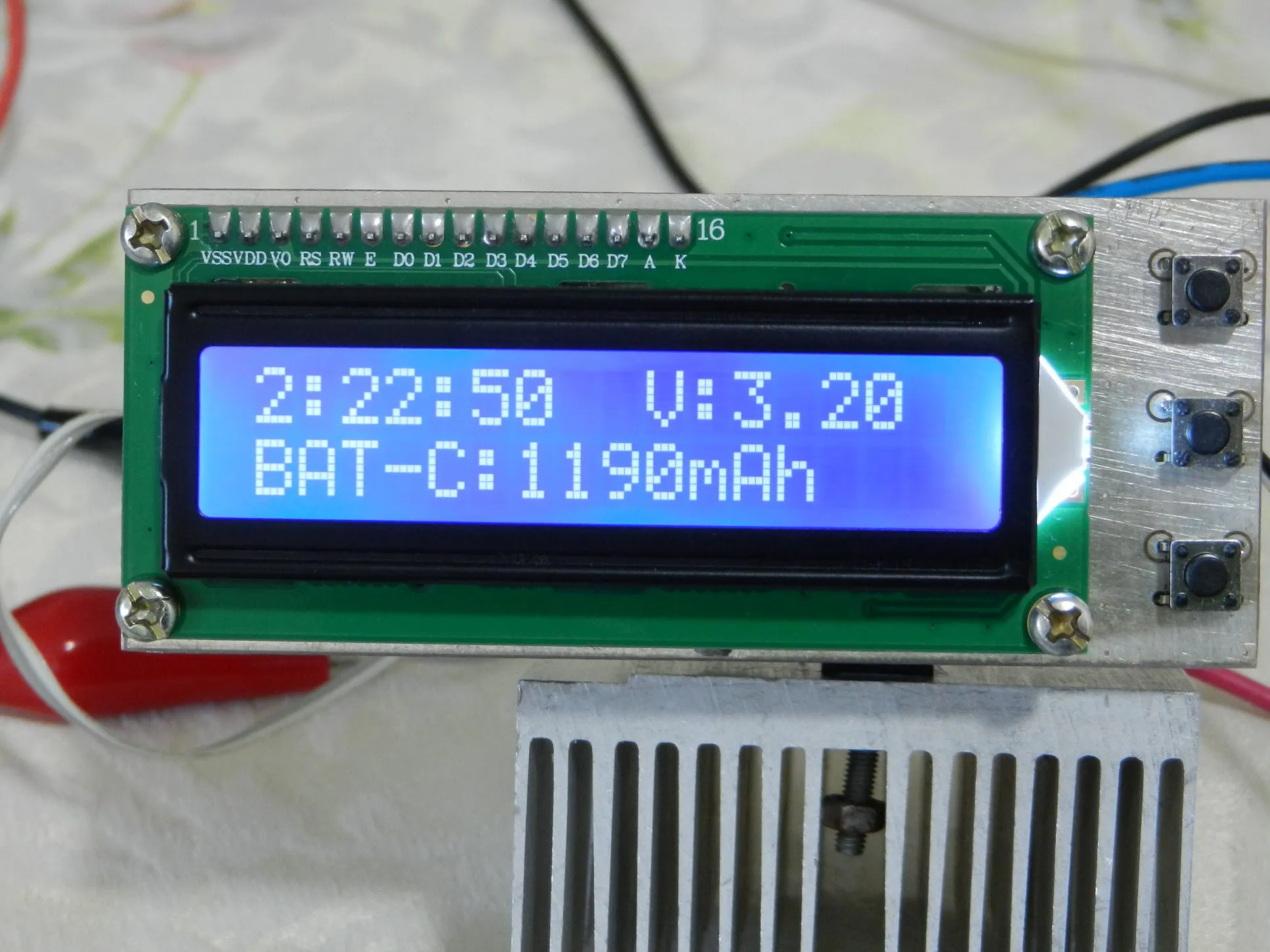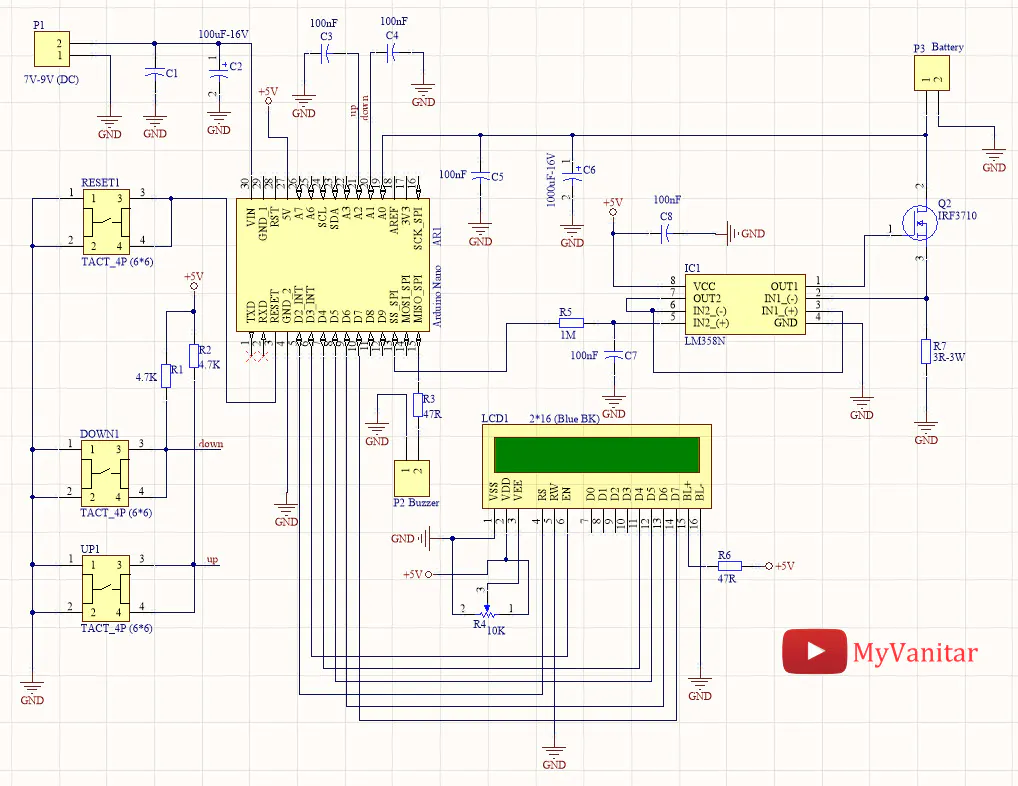# Measuring the Battery Capacity [Lithium-NiMH-NiCd]

Battery capacity measurement using Arduino [Lithium-NiMH-NiCd].

IntermediateFull instructions provided2 hours3,985## Things used in this project

### Hardware components

 Arduino Nano
×1

## Schematics## Code

### Code

C/C++
```#include <LiquidCrystal.h>
#include <JC_Button.h>

const float Low_BAT_level = 3.2;

//Current steps with a 3R load (R7)
const int Current[] = {0, 37, 70, 103, 136, 169, 202, 235, 268, 301, 334, 367, 400, 440, 470, 500, 540};

const byte RS = 2, EN = 3, D4 = 4, D5 = 5, D6 = 6, D7 = 7;
const byte PWM_Pin = 10;
const byte Speaker = 12;
const int BAT_Pin = A0;
int PWM_Value = 0;
unsigned long Capacity = 0;
float BAT_Voltage = 0;
byte Hour = 0, Minute = 0, Second = 0;
bool calc = false, Done = false;

LiquidCrystal lcd(RS, EN, D4, D5, D6, D7);

Button UP_Button(16, 25, false, true);
Button Down_Button(15, 25, false, true);

void setup() {

pinMode(PWM_Pin, OUTPUT);
pinMode(Speaker, OUTPUT);

analogWrite(PWM_Pin, PWM_Value);

UP_Button.begin();
Down_Button.begin();

lcd.setCursor(0, 0);
lcd.begin(16, 2);
lcd.print("Battery Capacity");
lcd.setCursor(0, 1);
lcd.print("Measurement v1.0");
delay(3000);
lcd.clear();
lcd.setCursor(0, 1);
lcd.print("0");

}

void loop() {

if (UP_Button.wasReleased() && PWM_Value < 80 && calc == false)
{
PWM_Value = PWM_Value + 5;
analogWrite(PWM_Pin, PWM_Value);
lcd.setCursor(0, 1);
lcd.print("     ");
lcd.setCursor(0, 1);
lcd.print(String(Current[PWM_Value / 5]) + "mA");
}

if (Down_Button.wasReleased() && PWM_Value > 1 && calc == false)
{
PWM_Value = PWM_Value - 5;
analogWrite(PWM_Pin, PWM_Value);
lcd.setCursor(0, 1);
lcd.print("     ");
lcd.setCursor(0, 1);
lcd.print(String(Current[PWM_Value / 5]) + "mA");
}

if (UP_Button.pressedFor(1000) && calc == false)
{
digitalWrite(Speaker, HIGH);
delay(100);
digitalWrite(Speaker, LOW);
lcd.clear();
timerInterrupt();
}

}

void timerInterrupt() {
calc = true;
while (Done == false) {
Second ++;
if (Second == 60) {
Second = 0;
Minute ++;
lcd.clear();
}
if (Minute == 60) {
Minute = 0;
Hour ++;
}
lcd.setCursor(0, 0);
lcd.print(String(Hour) + ":" + String(Minute) + ":" + String(Second));
lcd.setCursor(9, 0);
BAT_Voltage = ADC_Value * (5.0 / 1024);
lcd.print("V:" + String(BAT_Voltage));
lcd.setCursor(0, 1);
lcd.print("BAT-C: Wait!...");

if (BAT_Voltage < Low_BAT_level)
{
lcd.setCursor(0, 1);
lcd.print("                ");
lcd.setCursor(0, 1);
Capacity =  (Hour * 3600) + (Minute * 60) + Second;
Capacity = (Capacity * Current[PWM_Value / 5]) / 3600;
lcd.print("BAT-C:" + String(Capacity) + "mAh");
Done = true;
PWM_Value = 0;
analogWrite(PWM_Pin, PWM_Value);
digitalWrite(Speaker, HIGH);
delay(100);
digitalWrite(Speaker, LOW);
delay(100);
digitalWrite(Speaker, HIGH);
delay(100);
digitalWrite(Speaker, LOW);
}

delay(1000);
}
}
```